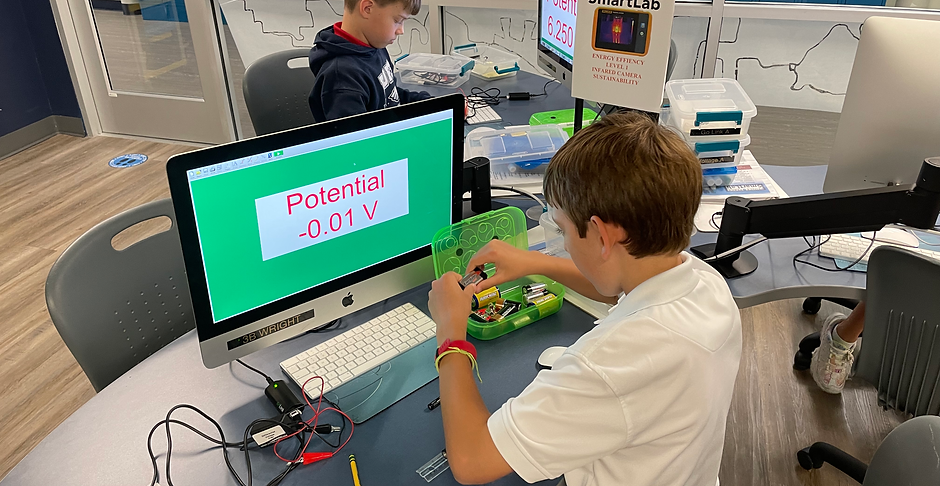top of page# Island 3 Solar Energy: Solar Photovoltaics with Vernier

Sustainability

POP students will continue to develop the "scientific method" and discover how to collect experimental data with digital sensors and software. Experiment with variable load and energy sensors that measure solar energy production## Solar Energy: Solar Photovoltaics with Vernier

How To Measure Solar Energy Output

### The light energy from the Sun, sent out in tiny packets of energy called photons, causes an electrical current to flow as soon as it is absorbed into the top surface of the PV cell. The secret of PV is in the chemistry of the semiconductor materials.

Current is the amount of electric charge flowing through a circuit and measured in amps. Current is a measure of the rate at which the electrons move past any point in a circuit. Voltage, also called potential, is the difference in electrical energy between two points. Voltage is measured in volts.

### Measuring Power Production

The amount of energy in electricity is a combination of current and voltage.

Power = Volts x Current

In science, “power”, means the amount of energy used per unit time.  The unit of measure for power is the Watt (W). You may recognize the word, “Watt”, from light bulbs. Bulbs come in various wattages like 60 W, 75 W, and 100 W. The number explains how much energy each bulb uses. A 100 W bulb uses 100 Joules (J), or units of energy, per second.

As a comparison, when you walk at a comfortable steady pace, you use a power of about 200 W, or 200 J/s. This means that you use up energy at about the same rate as two 100 W light bulbs.

bottom of page